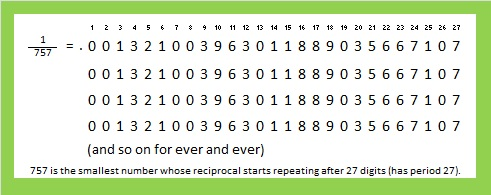# 757 and Level 4

• 757 is a prime number.
• Prime factorization: 757 is prime.
• The exponent of prime number 757 is 1. Adding 1 to that exponent we get (1 + 1) = 2. Therefore 757 has exactly 2 factors.
• Factors of 757: 1, 757
• Factor pairs: 757 = 1 x 757
• 757 has no square factors that allow its square root to be simplified. √757 ≈ 27.51363.How do we know that 757 is a prime number? If 757 were not a prime number, then it would be divisible by at least one prime number less than or equal to √757 ≈ 27.5. Since 757 cannot be divided evenly by 2, 3, 5, 7, 11, 13, 17, 19, or 23, we know that 757 is a prime number.

Here’s today’s puzzle. A logical way to solve it can be found in a table at the end of the post.Print the puzzles or type the solution on this excel file: 12 Factors 2016-01-25

—————————————

Here’s another way we know that 757 is a prime number: Since  its last two digits divided by 4 leave a remainder of 1, and 26² + 9² = 757 with 26 and 9 having no common prime factors, 757 will be prime unless it is divisible by a prime number Pythagorean hypotenuse less than or equal to √757 ≈ 27.5. Since 757 is not divisible by 5, 13, or 17, we know that 757 is a prime number.

757 is prime so the only way it can be written as the sum of consecutive numbers is 378 + 379 = 757.

757 is the sum of seven consecutive prime numbers: 97 + 101 + 103 + 107 + 109 + 113 + 127 = 757.

From Stetson.edu we learn this distinguishing fact about the number 757:Here are some square facts about the number 757:

26² + 9² = 757, and 468² + 595² = 757². That primitive Pythagorean triple, 468-595-757, can be calculated from 2(26)(9), 26² – 9², 26² + 9².

757 is also the sum of three squares two different ways:

• 24² + 10² + 9² = 757
• 18² + 17² + 12² = 757

Finally 757 is a palindrome in four different bases:

• 1001001 BASE 3; note that 1(3^6) + 0(3^5) + 0(3^4) + 1(3^3) + 0(3^2) + 0(3^1) + 1(3^0) = 757.
• 757 BASE 10; note that 7(100) + 5(10) + 7(1) = 757.
• 1F1 BASE 21 (F is 15 base 10); note that 1(21²) + 15(21) + 1(1) = 757.
• 111 BASE 27; note that 1(27²) + 1(27) + 1(1) = 757.

—————————————1.Paula Beardell Krieg